Fenrier Lab

# Delaunay 网格划分算法

### 定义

Delaunay 网格划分不是一种具体的算法，而是一种规范，它确保了网格中的三角形的形状处于可控的状态。这是网格划分中不得不考虑的问题，如果同一个单元中的节点离得老远，那么在插值的时候，其误差便没有保证，因为我们知道插值算法的精度依赖于距离。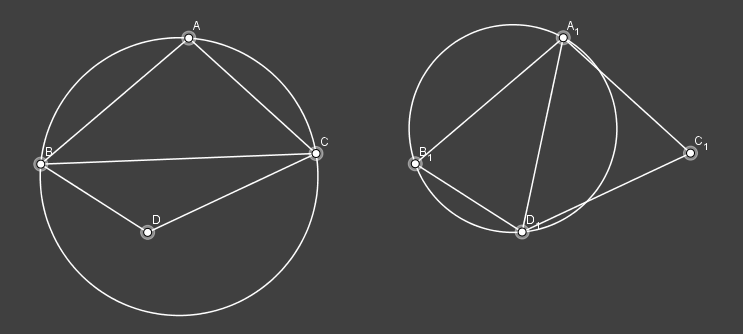### 平面点集的网格划分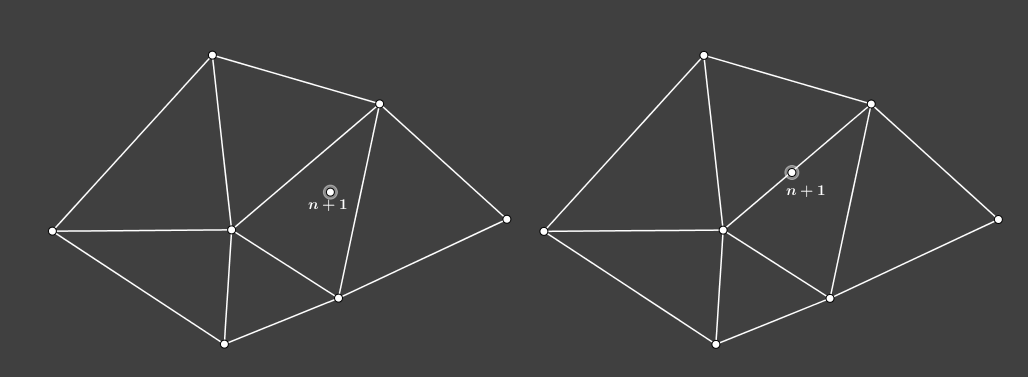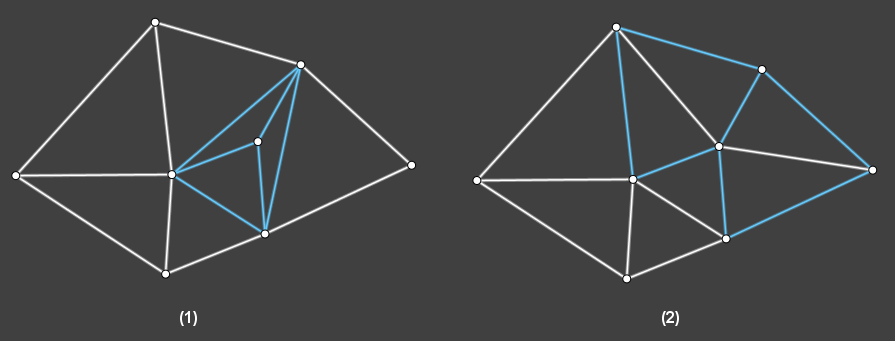### 关于初始化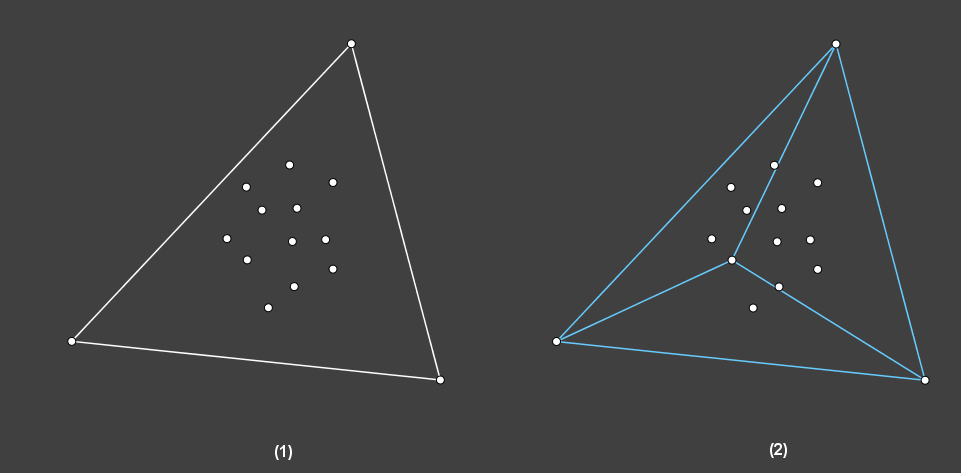### 数据结构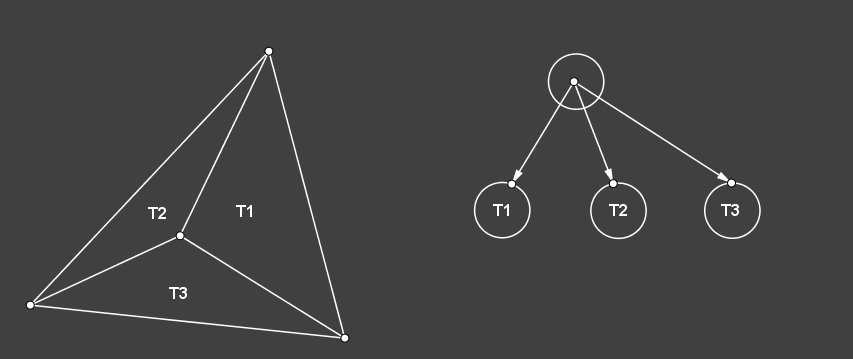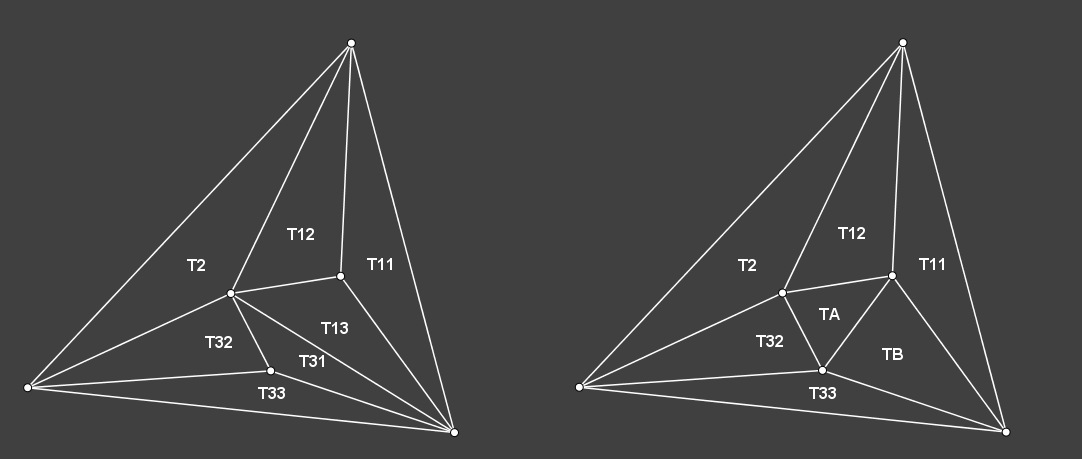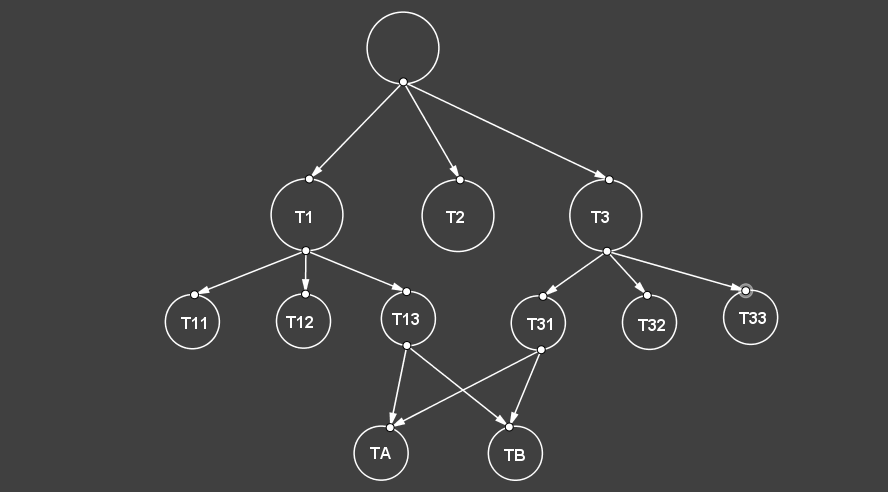triangles:

Index v1 v2 v3 flag
0 0 1 2
1 1 2 3
2 1 2 4

vertices:

Index x y
0 0 0
1 10 0
2 0 10

edges:

Index v1 v2 t1 t2 flag
0 0 1 0 1
1 1 2 1 2
2 2 3 3 3

### 非法边检测

$A(a_x, a_y),\quad B(b_x, b_y),\quad C(c_x, c_y),\quad D(d_x, d_y)$

$x = (c_1 * a_2 - c_2 * a_1) / (a_2 * b_1 - a_1 * b_2)\\ y = (c_1 * b_2 - c_2 * b_1) / (a_1 * b_2 - a_2 * b_1)$

\begin{aligned} a_1 &= b_x - a_x\\ b_1 &= b_y - a_y\\ c_1 &= \frac 1 2 (b_x^2 - a_x^2 + b_y^2 - a_y^2)\\ a_2 &= c_x - b_x\\ b_2 &= c_y - b_y\\ c_1 &= \frac 1 2 (c_x^2 - b_x^2 + c_y^2 - b_y^2) \end{aligned}